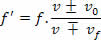Sound and Oscillation

# Doppler effect

The Doppler Effect is a sound effect that is named after the Austrian physicist Christian Johann Doppler, the first to analyze it.
Have you ever come across a situation where you heard the sound of an ambulance siren passing by? You may have noticed that the sound of an ambulance siren, or a fire engine, seems to be higher when the mobile (ambulance or fire engine) approaches than when it is moving away. In reality, the frequency emitted by the siren is always the same, what changes is our auditory sensation .

Let’s consider the situation represented in the figure above. The ambulance is stopped, but the siren is on. Observers A and B hear exactly the same frequency emitted by the ambulance siren. In the situation in the figure below, the frequencies heard by A and B will be different.

At the rear of the vehicle, compressions are farther apart, so A’s ear receives fewer compressions per second. In front of the ambulance, the compressions are closer together and thus observer B’s ear receives more compressions per second. Where f is the frequency emitted by the siren, A the frequency heard by A and B the frequency heard by B, we have:

A < f f B > f

In the figure above, we represent the sound source (ambulance) in motion and the observers at rest. There may also be the opposite case: source at rest and observer in motion . We can also have a more general situation, where both the source and the observers are in motion.

In all these cases, the frequency heard is different from the frequency emitted by the source, and this phenomenon is called the Doppler effect , after the Austrian physicist Christian Johann Doppler, the first to analyze it. If f is the frequency emitted by the source and f’ is the frequency heard by the observer, it can be shown that:On what:

v – speed of sound

0 – observer speed

f – speed of the sound source

In this equation, the higher signals must be used when there is approximation between source and observer , and lower signals must be used in case there is distance between source and observer .